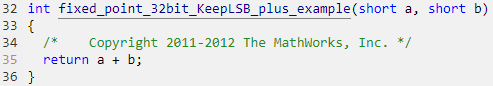# setfimath

Attach `fimath` object to `fi` object

## Syntax

``Y = setfimath(X,F)``

## Description

example

````Y = setfimath(X,F)` returns a `fi` object `Y` with `X`’s `numerictype` and value, and attached `fimath` object `F`.The `Y = setfimath(X,F)` syntax does not modify the input `X`. To modify `X`, use ```X = setfimath(X,F)```. This usage gives you more localized control over the `fimath` settings without making a data copy in the generated code. If you use `setfimath` in an expression, such as `a*setfimath(b,F)`, the `fimath` object is used in the temporary variable, but `b` is not modified.This function and the related `removefimath` function are useful for preventing errors about the `fimath` of both operands needing to be equal.```

## Examples

collapse all

Create a `fi` object.

`a = fi(pi)`
```a = 3.1416 DataTypeMode: Fixed-point: binary point scaling Signedness: Signed WordLength: 16 FractionLength: 13 ```

Create a `fimath` object and use `setfimath` to attach it to the `fi` object.

```f = fimath('OverflowAction','Wrap','RoundingMethod','Floor'); b = setfimath(a,f)```
```b = 3.1416 DataTypeMode: Fixed-point: binary point scaling Signedness: Signed WordLength: 16 FractionLength: 13 RoundingMethod: Floor OverflowAction: Wrap ProductMode: FullPrecision SumMode: FullPrecision ```

This example shows how to use the pattern `X = setfimath(X,F)` and `Y = removefimath(Y)` to insulate variables from `fimath` settings outside the function. This pattern does not create copies of the data in generated code.

`type fixed_point_32bit_KeepLSB_plus_example.m`
```function y = fixed_point_32bit_KeepLSB_plus_example(a,b) f = fimath('RoundingMethod', 'Floor', ... 'OverflowAction', 'Wrap', ... 'SumMode', 'KeepLSB', ... 'SumWordLength', 32) a = setfimath(a,f); b = setfimath(b,f); y = a + b; y = removefimath(y); end ```
```a = fi(0,1,16,15); b = fi(0,1,16,15);```

You can use MATLAB® Coder™ to generate C code. This example generates C code on a computer with a 32-bit native integer type.

```codegen -config:lib fixed_point_32bit_KeepLSB_plus_example... -args {a,b} -launchreport Code generation successful: View report ```

Trace the code in the code generation report.## Input Arguments

collapse all

Input array, specified as a scalar, vector, matrix, or multidimensional array.

If `X` is a `fi` object or integer data type, then the `fimath` object is applied. Otherwise, the `fimath` object is not applied and `Y = X`.

Data Types: `single` | `double` | `int8` | `int16` | `int32` | `int64` | `uint8` | `uint16` | `uint32` | `uint64` | `fi`
Complex Number Support: Yes

Fixed-point math settings to attach to the output, specified as an existing `fimath` object. If `F` is not a `fimath` object, an error occurs.

## Output Arguments

collapse all

Output `fi` object, returned as a `fi` object with the same data type and value as the input `X` and the attached `fimath` object `F`.

If the input `X` is not a `fi` object or integer data type, then `Y = X`.

## Version History

Introduced in R2012b